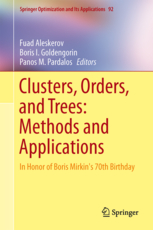• A
• A
• A
• АБB
• АБB
• АБB
• А
• А
• А
• А
• А
Обычная версия сайта

## The manipulability index in the IANC model

P. 391-404.

Procedures aggregating individual preferences into a collective choice differ in their vulnerability to manipulations. To measure it, one may consider the share of preference profiles where manipulation is possible in the total number of profiles, which is called Nitzan-Kelly's index of manipulability. The problem of manipulability can be considered in different probability models. There are three models based on anonymity and neutrality: impartial culture model (IC), impartial anonymous culture model (IAC), and impartial anonymous and neutral culture model (IANC). In contrast to the first two models, the IANC model, which is based on anonymity and neutrality axioms, has not been widely studied. In addition, there were no attempts to derive the difference of probabilities (such as Nitzan-Kelly's index) in IC and IANC analytically. We solve this problem and show in which cases the upper bound of this difference is high enough, and in which cases it is almost zero. These results enable us to simplify the computation of indices.

### В книгеПод науч. редакцией: F. T. Aleskerov, B. I. Goldengorin, P. M. Pardalos. Vol. 92. Berlin: Springer, 2014.